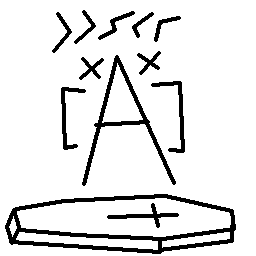#Coffee Space

Listen:

## Array Pointer DecayPreview Image

### TL;DR

Arrays are weird and `sizeof()` may not always work how you expect. An array is not the same as a pointer and sometimes you will come unstuck. There are several C-based solutions to this problem depending on your specific needs, each with it's role to play.

### Problem

So we have some array, let's say `s` - and we want to determine the size in C. A pretty standard thing to do. So let's do it:

``````int main(){
char s[] = {"Hello World!"};
printf("Size of 's' is %u\n", sizeof(s));
}``````

This then outputs `Size of 's' is 13`, so all is good. But we don't plan to use it here, we plan to use it in some function, like so:

``````void test(char* s){
printf("Size of 's' is %u\n", sizeof(s));
}

int main(){
char s[] = {"Hello World!"};
test(s);
}``````

The output is now `Size of 's' is 8`. Hang on a second! What? `sizeof()` now fails to return the correct size because I passed it to a function? That "8" seems familiar some how... It doesn't feel like a coincidence that it is the same size as a pointer...

This isn't the first time I've run into this, my previous solution was to supply the length as an additional parameter, curl up into a ball and forget the problem exists. But not this time. This time I will grapple with the oddity (because I'm putting off doing more important work).

### What?

Okay, so apparently it is some kind of array pointer decay. I never formally got taught C or C++, so this was interesting to learn of indeed. I was under the impression that things wouldn't do strange things in C, this is why I use it after all. But it turns out, this is not so much the case. Why do you do this to me?!

It turns out an array is a special thing, you can declare:

``char s[] = {"Hello World!"};``

And this is a proper array. But if I pass this to a function normally (by pointer), information about size is lost.

It turns out there are several representations of the array:

• `char* a` - By pointer.
• `char a[]` - By value.
• NOTE: In C++, you can also do this by reference: `char (&a)[t]`

Without going into details that others have, only functions that receive arrays by reference can use `sizeof()` to determine array size.

### Potential Solutions

So here we have a comparison of potential solutions (including the ones that don't work):

``````void test_ptr(char* a){
printf("(ptr) Size of 'a' is %u\n", sizeof(a));
}

void test_val(char a[]){
printf("(val) Size of 'a' is %u\n", sizeof(a));
}

struct Structure{
char* a;
unsigned int len;
};

void test_str(struct Structure s){
printf("(str) Size of 'a' is %u\n", s.len);
}

void test_cnt(char* a){
int len = 0;
while(a[len++] != '\0');
printf("(cnt) Size of 'a' is %u\n", len);
}

void test_par(char* a, int len){
printf("(par) Size of 'a' is %u\n", len);
}

int main(){
char s[] = {"Hello World!"};
printf("Size of 's' is %u\n", sizeof(s));
// by pointer
test_ptr(s);
// by value
test_val(s);
// structure method
struct Structure z;
z.a = s;
z.len = sizeof(s);
test_str(z);
// count method
test_cnt(s);
// param method
test_par(s, sizeof(s));
}``````

This results in:

``````Size of 's' is 13
(ptr) Size of 'a' is 8
(val) Size of 'a' is 8
(str) Size of 'a' is 13
(cnt) Size of 'a' is 13
(par) Size of 'a' is 13``````

The counting method (`test_cnt()`) is really only appropriate for simple array types that you know their terminating byte. This is not reliable and is quite slow.

The structure method (`test_str()`) is fine enough, but it could add complexity to your code, when all you really want is a simple array. Inventing data types is all fine and dandy until your code grows and is used by others.

The parameter method (`test_par()`) is probably ideal, as long as the number of parameters you have is quite small. This is the one I have been using for years as a workaround anyway.